# The rating of the best interest rates under the term deposits for 1 month30.06.2020

## Bankchart.com.au has surveyed the terms of term deposits for 1 month in the national currency. The survey covered all the deposits with any form of interests repayment, regardless of the replenishment option or the deposit partial withdrawal option. Our website's analysts have studied the loan offers from all Australian banks. Review date - 30.06.2020

As of 23 June 2020 the deposits under review have been offered by 24 banks.  The average interest rate under such deposits equals 0,37 % p.a. The total number of the deposits programs available for the specified terms is 50.

The maximal return among the deposits under review is offered by Arab Bank. The interest rate under "Fixed Term Deposit" deposit program constitutes 1,10 % p.a. The table below shows the best offers by each bank selected by Bankchart.com.au (in terms of profitability) for the aforementioned terms.

### The TOP-24 term deposits for 1 month, as of 23 June 2020

#

Name

Interest Rate, %

1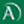Arab Bank

«Fixed Term Deposit»

1,10

interest rate, %

rate type

fixed

interest periodicity

at maturity

minimum sum

\$ 50 000,00

2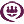RACQ

«Term Deposit»

1,00

interest rate, %

rate type

fixed

interest periodicity

monthly

minimum sum

\$ 1 000,00

3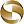Mega International Commercial Bank

«Term Deposits»

0,85

interest rate, %

rate type

fixed

interest periodicity

at maturity

minimum sum

\$ 1 000,00

4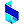Bank of Sydney

«Online Term Deposit»

0,85

interest rate, %

rate type

fixed

interest periodicity

at maturity

minimum sum

\$ 50 000,00

5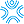Bank First

«Term Deposits»

0,75

interest rate, %

rate type

fixed

interest periodicity

monthly

minimum sum

\$ 500,00

6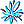AMP Bank

«Term Deposits»

0,70

interest rate, %

rate type

fixed

interest periodicity

at maturity

minimum sum

\$ 500 000,01

7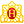Heritage Bank

«Term Deposits»

0,55

interest rate, %

rate type

fixed

interest periodicity

at maturity

minimum sum

\$ 1 000,00

8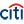Citibank

«Term deposit»

0,50

interest rate, %

rate type

fixed

interest periodicity

monthly

minimum sum

\$ 75 000,00

9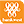Bank of Western Australia

«Online Term Deposit»

0,45

interest rate, %

rate type

fixed

interest periodicity

at maturity, annually

minimum sum

\$ 5 000,00

10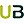Ubank

«Term deposit»

0,40

interest rate, %

rate type

variable

interest periodicity

at maturity

minimum sum

\$ 0,00

11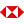HSBC Bank

«Term Deposits»

0,35

interest rate, %

rate type

fixed

interest periodicity

at maturity

minimum sum

\$ 5 000,00

12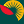Suncorp Bank

«Term Deposit»

0,35

interest rate, %

rate type

fixed

interest periodicity

at maturity

minimum sum

\$ 1 000 000,00

13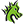St. George Bank

«Term Deposits»

0,30

interest rate, %

rate type

fixed

interest periodicity

at maturity

minimum sum

\$ 1 000,00

14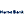Hume Bank

«Term Deposits»

0,30

interest rate, %

rate type

fixed

interest periodicity

at maturity

minimum sum

\$ 5 000,00

15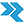Rural Bank

«Short Term Deposit»

0,25

interest rate, %

rate type

fixed

interest periodicity

monthly

minimum sum

\$ 50 000,00

16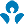Australia and New Zealand (ANZ)

0,25

interest rate, %

rate type

fixed

interest periodicity

at maturity

minimum sum

\$ 5 000,00

17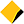Commonwealth Bank of Australia

«Term Deposits»

0,20

interest rate, %

rate type

fixed

interest periodicity

monthly, at maturity, every 6 months

minimum sum

\$ 5 000,00

18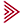Bendigo and Adelaide Bank

«Term deposit»

0,20

interest rate, %

rate type

fixed

interest periodicity

at maturity

minimum sum

\$ 5 000,00

19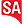Bank SA

«Term Deposits»

0,20

interest rate, %

rate type

fixed

interest periodicity

at maturity

minimum sum

\$ 1 000,00

20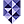Bank of Melbourne

«Term Deposits»

0,20

interest rate, %

rate type

fixed

interest periodicity

at maturity

minimum sum

\$ 1 000,00

21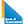Bank of Queensland

«Term deposit»

0,20

interest rate, %

rate type

fixed

interest periodicity

at maturity

minimum sum

\$ 5 000,00

22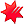National Australia Bank

«NAB Term Deposits»

0,20

interest rate, %

rate type

fixed

interest periodicity

at maturity

minimum sum

\$ 5 000,00

23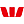Westpac Bank

«Term Deposit»

0,15

interest rate, %

rate type

fixed

interest periodicity

monthly

minimum sum

\$ 5 000,00

24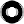Macquarie Bank

«Term Deposit»

0,10

interest rate, %

rate type

fixed

interest periodicity

monthly

minimum sum

\$ 0,00

To view the up-to-date rating of the deposits at our website here.